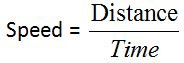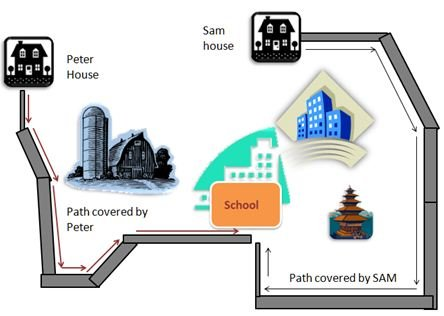# Definition Of Speed

## Definition Of Speed

The distance moved by an object in a specific time" is known as speedThe path covered by a body/ object in a given time is called speed of the object
In S.I system speed is measured in m/s ( Meters / Second ).
Distance is measured in meters and time in seconds hence m/s is the unit for speed.
For measuring distance, direction is not necessary (path can be measured in any direction) hence speed is not represented by any specific direction.
Speed of an object increases when a body covers a long path in short interval of time.
Speed of on object decreases when an object covers a short (small) path in long duration of time.
Example: Peter and Sam go to the school on their own bicycles. Peter’s house is about 1500 meters from the school. Sam’s house is about 2400 meters from the school. Peter takes about 5 minutes in riding from house to school and Sam takes 6 minutes in riding from his house to school. Who is riding the bicycle with more speed?Distance covered by the Peter = 1500 meters
Time taken by Peter to cover the distance = 5 minutes.
First convert the time into seconds.
1 minute = 60 seconds
5 minutes = 60 x 5 = 300 seconds
Speed = Distance / Time = 1500 / 300 = 5 m/s ( ∴Peter’s speed = 5 m/s )
Distance covered by Sam = 2400 meters.
Time taken by Sam to cover the distance = 6 minutes.
First convert the time into seconds.
1minute = 60 seconds
6 minutes = 60 x 6 = 360 seconds
Speed = Distance / Time = 2400 / 360 = 240 / 36 = 20 / 3 = 6.33 m/s
Sam is riding the bicycle with more speed than Peter.